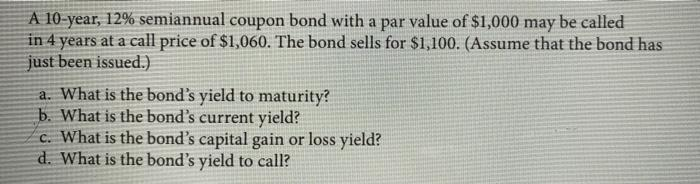# Question Solved1 Answer A 10-year, 12% semiannual coupon bond with a par value of \$1,000 may be called in 4 years at a call price of \$1,060. The bond sells for \$1,100. (Assume that the bond has just been issued.) a. What is the bond's yield to maturity? b. What is the bond's current yield? c. What is the bond's capital gain or loss yield? d. What is the bond's yield to call? A 10-year, 12% semiannual coupon bond with a par value of \$1,000 may be called in 4 years at a call price of \$1,060. The bond sells for \$1,100. (Assume that the bond has just been issued.) a. What is the bond's yield to maturity? b. What is the bond's current yield? c. What is the bond's capital gain or loss yield? d. What is the bond's yield to call?Transcribed Image Text: A 10-year, 12% semiannual coupon bond with a par value of \$1,000 may be called in 4 years at a call price of \$1,060. The bond sells for \$1,100. (Assume that the bond has just been issued.) a. What is the bond's yield to maturity? b. What is the bond's current yield? c. What is the bond's capital gain or loss yield? d. What is the bond's yield to call?
More
Transcribed Image Text: A 10-year, 12% semiannual coupon bond with a par value of \$1,000 may be called in 4 years at a call price of \$1,060. The bond sells for \$1,100. (Assume that the bond has just been issued.) a. What is the bond's yield to maturity? b. What is the bond's current yield? c. What is the bond's capital gain or loss yield? d. What is the bond's yield to call?